#beanz Magazine

## Variables, Constants, and Data Types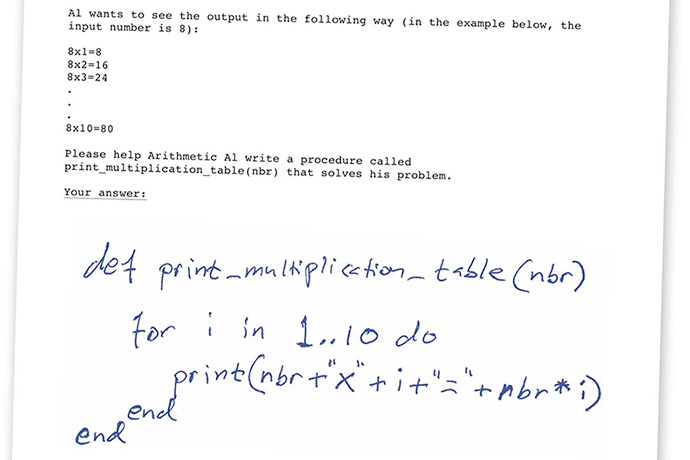Learn without a computer or programming language. Includes exercises.

Editor’s Note: I recently took a class, Pen and Paper Coding, which teaches programming with pen and paper instead of computers and a specific language. Erik Linde, the founder and teacher, let me reprint one part of a class so you can experience this remarkable computer-free and language-free way to learn programming. This article — which has been modified from its original — teaches variables, constants, and data types with exercises you can do to test your knowledge.

As we begin writing real code, one of the first things we must familiarize ourselves with is variables, and their close cousin, constants.

A variable, according to its name, is something that allows its value to vary. In programming, we use variables to store data while we run our programs.

A variable can be contrasted with a constant, whose value is not allowed to change. In programming, we use constants to store information that we know is never going to change.

Even though variables and constants may sound very different from each other semantically, in reality, they are quite similar.

We will start with studying constants before we look at variables, as variables will be easier to understand once we have understood constants.

### Constants

My name is Erik, and I think it’s fair to say that that is a constant, as it will never ever change. The absolute zero temperature, is also a constant at -273 — it will never change either. The number of seconds in one hour is a constant as well; it will never change: there will always be 3,600 seconds in an hour.

A constant — for the purposes of computer programming — consists of two things: a name, and a value. The name should clearly illustrate what the constant is all about, and be more or less self-explanatory. The value is the actual value of the constant (for example, -273 for absolute zero, and 3,600 for the number of seconds in an hour).

In this programming book, we will name our constants with uppercase letters. This allows us to clearly identify them as constants as soon as we run into them in our code, and thus be able to distinguish them from variables (which are written using lower case letters).

A constant is created using the const reserved word, followed by the constant’s data type, followed by the constant’s name, and finally, followed by an = sign and a value.

Let’s create a few constants so that we can see what they look like. What would be appropriate names for the constants in the examples above? Names for variables and constants are always up to the programmer, but here are some suggestions:

```const int ABSOLUTE_ZERO_TEMP = -273 const uint NBR_SECONDS_PER_HOUR = 3600```

For now, don’t worry about the meaning of int or uint. They’re data types and we’ll explain it later.

When picking names for constants and variables, we want to be expressive in order to reduce the risk of ambiguity, but it is also absolutely fine to use abbreviations for long names, so that they don’t take up the whole screen. For example, “NBR” or “nbr” is a common abbreviation for “number,” and one you will see a lot.

There is another rule when we pick names: the names must only consist of alphanumerical characters, i.e. A-Z and 0-9, as well as the underscore (_). You can use the underscore to separate words from each other so that they become more legible.

#### Initialization

In programming terms, what we just did above was to initialize our two constants. When we initialize a constant (or variable), we do two things at once:

1. We declare the constant: i.e. we inform the computer about our intention to use the constant or variable in the program, and we give it a name and a data type so that our computer can allocate memory for it and be ready for when we actually use it.
2. We assign the constant a value. For constants, that value can never be changed. Once we run our
program, i.e. once our computer starts executing the code, the constant has been locked in and can not be changed. Once the execution terminates, we can go back and change the constant’s value in our code, and re-run the program again for a different outcome.

#### Why would we need constants?

Constants are very useful when we want to set a value once, and then repeat it in many locations inside our program. If we didn’t use constants, but rather wrote out -273 in several different locations in our program (wherever we wanted to refer to absolute zero), and we ever wanted to change the -273 to a different number, we would have to change it in multiple locations, rather than just one. According to Murphy’s law, the saying that anything that can go wrong will go wrong. This is usually a recipe for disaster because, more often than not, even if we forgot to change only one of the values, it could lead to strange errors when we run our program.

By declaring a constant once and then re-using it, that allows us to easily change the value of the constant each time we run the program, and we only have to change your code in one location — as opposed to many — which reduces the risk of introducing errors into our code.

### Variables

Variables are similar to constants, but the main difference is that while a constant can not change once you have assigned it a value, a variable can, and oftentimes does.

We define a variable in a similar fashion as we define a constant, but instead of using the reserved word const, we use var.

#### Declaration

Let’s introduce a concept called declaration. Declaration can be thought of as the same as initialization, but without giving the variable a value. When we declare a variable, we simply inform the computer of our intention to use the variable in our program, so that the computer can allocate memory for it.

Sometimes we may prefer to not give our variables a value immediately, and in some languages, typically older languages, we are actually forced to declare all our variables in the beginning of the code, regardless of where in the code we intend to use them.

In more modern languages, best practices indicate that it is preferable to declare / initialize variables as close as
possible to their first use, so the concept of declaration is not as significant anymore as it used to be.
Still, for educational purposes, let’s declare a variable designed to store the current temperature:

`var int current_temperature`

That variable is now available for use in our code, and we can change its value as many times as we like. In fact, variable values are often changed many times in a program — sometimes even millions of times.

#### Why would we need variables?

Let’s say your programming teacher has asked you to write a program that simulates the rolling of a dice 10 times, and at the end sums up all the rolls and presents that number on the screen. Your teacher has instructed you to keep track of the progress using variables. How many variables might you need?

In this case, we would need one variable to keep track of the sum, and one to keep track of how many times we have rolled the dice. We might therefore start by initializing a variable dice_roll_sum to 0, because when we start the program, we have not yet rolled the dice, and hence its sum is 0:

`var uint dice_roll_sum = 0`

Remember that when we initialize a variable, we both declare it — give it a type and a name — as well as give it a value.

We would also initialize a variable nbr_dice_rolls to 0, as we haven’t yet rolled the dice.

`var uint nbr_dice_rolls = 0`

Each time our program “rolls the dice,” we would add the number displayed on the dice to the dice_roll_sum variable. After the first dice roll, dice_roll_sum might be 4, after the second it might be 9, after the third it might be 11, etc. The point is that dice_roll_sum will always keep track of the total sum of the rolls, just as its name indicates. Also, each time we rolled the dice, we would increment nbr_dice_rolls by 1, and then stop running our program when nbr_dice_rolls reached 10.

It should be noted that once the program stops running, all variables and constants and their respective values are lost. That’s why we will typically have our programs print information to the screen (or otherwise output or save
the results) while we run our programs so that we can preserve the results.

### Integers and Strings

#### Let’s talk about data types!

Just like we may be able to describe words in English as being of certain types (personal names, numbers, etc), we can do the same with variables and constants in a computer programming language.

For example, in English, 0, 3.1415, -2434 and 2*E6 might be classified as numbers, whereas Steve, Elon, Larry and Mark might be classified as names. Computers use similar types, but require a bit more more granularity.

We will therefore familiarize ourselves with two common data types in programming: integers and strings.

#### Integers

Integers are simply numbers without a decimal. Integer variables that can take on both negative and positive values are referred to as signed integers. Signed refers to the fact that they can have a minus sign in front of them. Integer variables that can only take on positive values are referred to as unsigned integers.

When we initialized dice_roll_sum above to 0, we wrote:

`var uint dice_roll_sum = 0`

We picked the unsigned integer as the type here (written as uint), as we know that our dice roll sum can never be negative. The reason for picking an unsigned integer is that, should we choose to roll the dice many, many times, an unsigned integer can contain twice as large a number as a signed integer. The explanation for this is simple: both a signed integer and an unsigned integer will be allocated the same space in memory; for a signed integer, however, half of its capacity will be below the minus sign, and half will be above — for the unsigned integer, all of its capacity will be above the minus sign.

Whether you declare an integer variable as signed or unsigned is up to you, and will depend on the circumstances, although most of the time, you will probably end up using signed integers just because those give you the most flexibility. When learning programming theory however, and when dealing with very large numbers, it is worth noting the difference between signed and the unsigned integers.

In the example below, we must use a signed integer (written as int), since we must allow for negative cash flows.
We initialize a signed integer variable in the following way:

`var int monthly_cash_flow = -50`

#### A few words on bits and computer memory

The bit is the smallest unit of information that can be stored in a computer’s memory. A bit can only take on one of two values: 0 or 1.

An integer variable is stored in memory using one or more bits; typically 32 or 64 bits in most modern computers.
The more bits allocated to an integer, the higher its largest value can be. The general formula for how many values
an integer can take on is 2^n, where n is equal to the number of bits. This means that a 1-bit integer can take on two (2^1) different values (0 and 1), a 2-bit integer can take on four (2^2) different values (0, 1, 2 and 3), and a 4-bit integer can take on 16 (2^4) values (0–15), etc.

#### Strings

The string is the second data type that we will get to know. Strings in programming can basically be said to equal text in plain English. Here are a few examples of strings (note that we will use the keyword string to indicate that we are declaring a string):

```var string student_name = "Pete" var string current_city = "New York" var string first_letter_in_alphabet = "A" var string plain_sentence = "Today is a great day!" var string sentence_with_number = "My phone number is 123 456 7890"```

All of the above variables are string variables, and all of them have been set to equal text surrounded by quotes.

The quotes are in fact one of the requirements of a string. Without the quotes, it would not be a string! Whether you use double quotes or single quotes is up to you, as they can be considered equivalent for the purposes of this book (i.e., “Pete” is equivalent to ‘Pete’).

#### Variables vs. literals

We now know how to properly refer to what’s to the left of the = sign — we have learned that those are called either variables or constants. This begs the obvious question: so far, what has then been to the right of the = sign? The answer is literals. In the example above, we say that “Pete” is a string literal, and -50 is an integer literal.

A literal is anything that is written directly into the code, for example, text and numbers. Literals can show up in many locations of your code, not only to the right of an = sign.

### Operations on Integers and Strings

Both integers and strings would be somewhat uninteresting if we couldn’t perform any operations on them. By operations we are, at this point, referring to arithmetics for integers (i.e. adding, subtracting, dividing or multiplying) and string concatenation for strings (i.e. joining two or more strings together).

#### Integer operations

A few examples of operations performed on integers will follow below:
We start by initializing two unsigned integers, with data type uint, numbers that won’t have a minus sign in front of them.

```var uint dollars_in_wallet = 30 const uint COST_OF_ICECREAM = 5```

We then purchase 3 ice creams, and reduce dollars_in_wallet accordingly! Note that we are simply applying basic algebra here.

`dollars_in_wallet = dollars_in_wallet - 3 * COST_OF_ICECREAM`

Our dollars_in_wallet variable now has the value 15. Let’s then say that we get lucky and find \$5 on the street, and then proceed to donate half of what we have in our wallet to a charity:

```dollars_in_wallet = dollars_in_wallet + 5 dollars_in_wallet = dollars_in_wallet / 2```

Our dollars_in_wallet variable now has the value 10.

#### String operations

Let’s turn our attention to strings operations now. In this section, we will only discuss concatenation. Concatenation is the act of combining two or more strings. For example, if you concatenated the two strings “Programming ” and “class,” you would end up with “Programming class”.

Here is how we concatenate two strings:

```var string first_name = "Erik" var string last_name = "Linde" var string full_name = first_name + " " + last_name```

full_name now has the value “Erik Linde”. As you can see, we simply use the + sign when we want to concatenate strings. Observe the empty space we inserted between the two strings.

### Exercises

1. Explain in plain English what a variable is and is not.

2. Explain in plain English what a constant is and is not.

3. Declare an integer variable named dice_roll_sum, designed to hold very large, strictly positive numbers (please pick the most appropriate data type if more than one type is possible).

4. Declare an integer variable named quarterly_income, designed to hold both negative and positive numbers.

5. Initialize a constant named ABSOLUTE_ZERO_TEMP to -273.

6. Initialize four variables: q1_income, q2_income, q3_income and q4_income to 200, -50, 100 and 0 respectively. Then create a new variable, annual_income, which you set to equal the sum of all of the above variables. In the end, please also write down the value of annual_income.

7. Write down the ending value of x.

```var int x = 5 var int y = 3 x = x + 5 x = x - y```

8. Write down the ending value of z.

```var int x = 10 var int y = 3 var int z = 4 * x + y```

9. Write down the ending value of z.

```var int x = 10 / 3 var int y = x * 2 var int z = (y - x) / x x = z * x * y```

10. ADVANCED What mistakes can you find in the code below?

```var int x = 1 var int y = x var int z = x + y var int x = z + x + y var int q = q + x```

11. Explain, in plain English, what string concatenation is.

12. Please write down the ending value of the variable result:

```var string a = "The" var string b = " " var string c = "fantas" var string d = "tic" var string e = "4" var string result = a + b + c + d + b + e```

13. ADVANCED Please initialize a new string variable named phone_nbr and set its value to equal “+1 (212) 123 4567” without using any numbers anywhere in your code:

```var string country_code = "1" var string area_code = "212" var string local_nbr = "123 4567"```

1. A variable is a stored value whose data might vary based upon how and where it is used in a computer program.

2. A constant is a stored value whose data does not vary, regardless of where it is used in a computer program.

3. Declare an integer variable named dice_roll_sum, designed to hold very large, strictly positive numbers (please pick the most appropriate data type if more than one type is possible).

`var uint dice_roll_sum`

The uint data type is most appropriate because the number of rolls will never be negative. Because values will always be positive, the data type should be unsigned. If you recall, the int data type can include values with a negative sign (signed) and positive (unsigned).

4. Declare an integer variable named quarterly_income, designed to hold both negative and positive numbers.

`var int quarterly_income`

5. Initialize a constant named ABSOLUTE_ZERO_TEMP to -273.

`const int ABSOLUTE_ZERO_TEMP = -273`

6. Initialize four variables: q1_income, q2_income, q3_income and q4_income to 200, -50, 100 and 0 respectively. Then create a new variable, annual_income, which you set to equal the sum of all of the above variables. In the end, please also write down the value of annual_income.

```var int q1_income = 200 var int q2_income = -50 var int q3_income = 100 var int q4_income = 0 var int annual_income = q1_income + q2_income + q3_income + q4_income```

When you substitute numbers for variables, annual_income is equal to 200 + -50 + 100 + 0 or 250.

7. Write down the ending value of x.

```var int x = 5 var int y = 3 x = x + 5 x = x - y```

When you substitute numbers for variables, the final value for x is 7. x = 5 + 5 or 10 and x = 10 – 3 or 7.

8. Write down the ending value of z.

```var int x = 10 var int y = 3 var int z = 4 * x + y```

When you substitute numbers for variables, the final value for z is 43. z = 4 * 10 + 3 or 43.

9. Write down the ending value of z.

```var int x = 10 / 3 var int y = x * 2 var int z = (y - x) / x x = z * x * y```

When you substitute numbers for variables, the final value for z is 1. The data type int does not store decimal data.

```x = 3 y = 3 * 2 [= 6] z = (6 - 3) / 3 [= 3 / 3 = 1]```

We don’t need to calculate the final value for x because we’ve been asked to find the value for variable z, not x. Paying attention is a critical skill for programmers.

10. ADVANCED What mistakes can you find in the code below?

```var int x = 1 var int y = x var int z = x + y var int x = z + x + y var int q = q + x```

The following things are wrong with this code:

In the fourth line, var int x = z + x + y, the variable x has already been initialized in the first line. Variables are initialized only once.

In the fifth line, var int q = q + x, you cannot declare a variable then use the variable in the assignment of a value to that variable.

11. String concatenation is the joining of data of string type in an order specified with code.

12. Please write down the ending value of the variable result:

```var string a = "The" var string b = " " var string c = "fantas" var string d = "tic" var string e = "4" var string result = a + b + c + d + b + e```

When you substitute the assigned string data for variables, the answer is The fantastic 4.

`result = "The" + " " + "fantas" + "tic" + " " + "4"`

Note the 4 is not an integer because it is between quote marks, signaling 4 is a string not an integer.

13.ADVANCED Please initialize a new string variable named phone_nbr and set its value to equal “+1 (212) 123 4567” without using any numbers anywhere in your code:

```var string country_code = "1" var string area_code = "212" var string local_nbr = "123 4567"```

``` ```

`var string phone_nbr = "+" + country_code + " (" + area_code + ") " + local_nbr`

It is important to include the blank space before the ( and after the ) to ensure the code prints out exactly as the example.

#### Pen and Paper Coding

https://www.penpapercoding.com

### Also In The April 2015 Issue### Why Do Designers Hate Comic Sans?

The history of the font and the reasons people hate Comic Sans are great ways to learn about typography.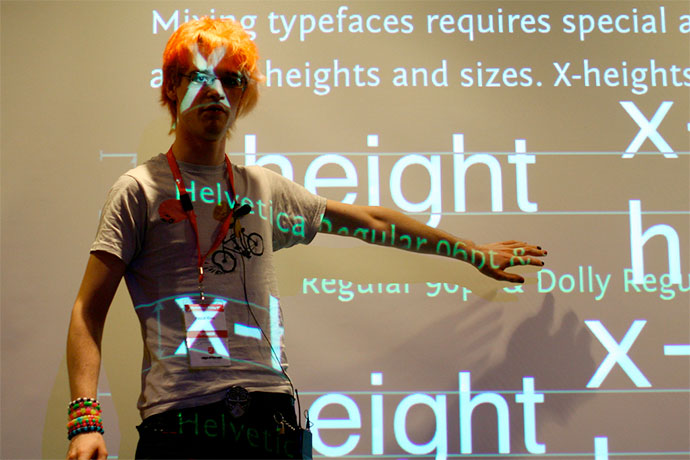### Learn Typography Online

Learn typography online and practice basic typography skills.### The 10 Principles of Good Web Design for Developers

Design basics for developers who do not have a designer around to say "no comic sans!"### Mary Glendening Talks about Library Makerspaces as Community Technology Centers

Librarian Mary Glendening describes how they created their library makerspace.### An Interview with Joe Dytrych and Dee Saigal, Creators of Erase All Kittens

Learn how two people created a fun online game to teach HTML and CSS.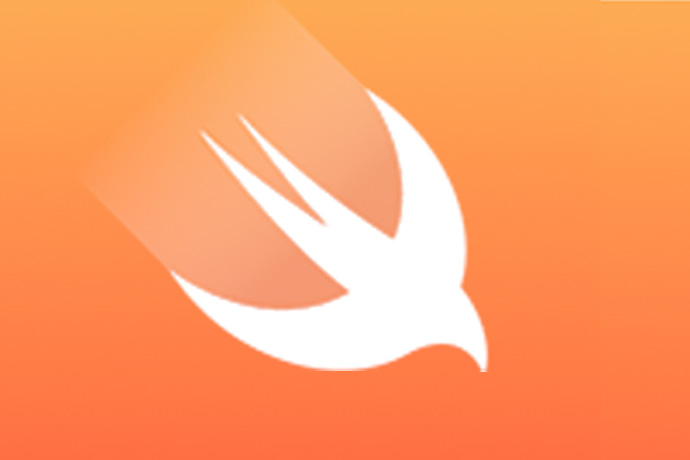### Swift

Apple's new Swift programming language is an interesting language to study and learn, plus an opportunity to watch a new language evolve.

### A designer is an emerging synthesis of artist, inventor, mechanic, objective economist and evolutionary strategist.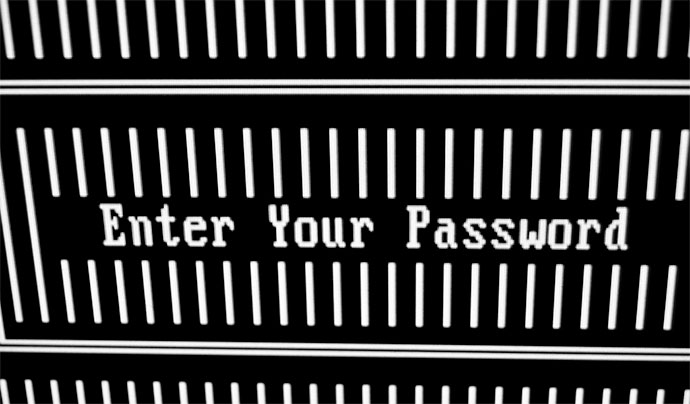A real life case study to explore online security, privacy, and the law.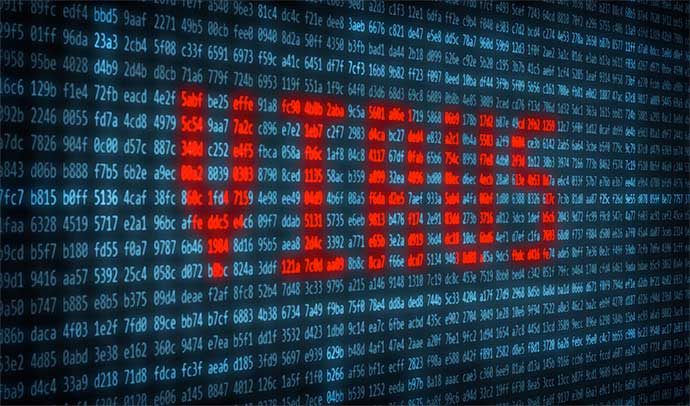### Online Security Tips

How to create secure passwords, manage your email accounts, securely backup data, and more.### April 2015 News Wire

Interesting stories about computer science, software programming, and technology.### Variables, Constants, and Data Types

Learn without a computer or programming language. Includes exercises.

Links from the bottom of all the April 2015 articles, collected in one place for you to print, share, or bookmark.### Code Golf

Problems software programmers can do to practice their skills, from beginner to advanced.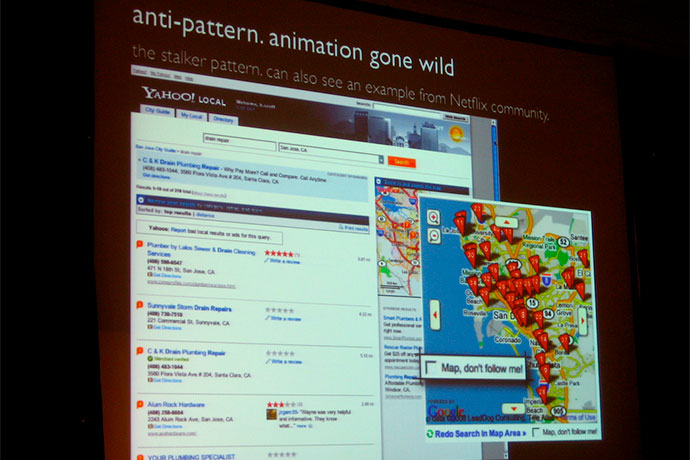### Design Patterns and Anti-Patterns

How programmers use design patterns and anti-patterns to develop software.### Help Build Applications Online

This website lets anyone practice their coding and design skills.